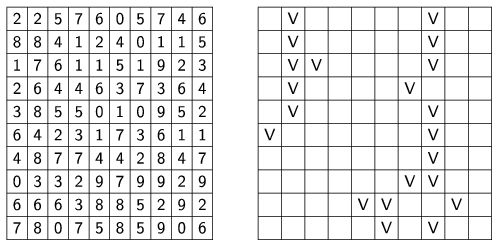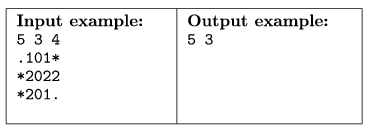Mathematical and Physical Journal
for High Schools
Issued by the MATFUND Foundation
 Already signed up? New to KöMaL?

# KöMaL Problems in Informatics, May 2007

Show/hide problems of signs:## Problems with sign 'I'

Deadline expired on June 15, 2007.

I. 160. One often finds incorrect HTML pages on the web, with incomplete commands as a typical error. Your program should check whether every <command> in the HTML file has a closing </command> tag. You do not need to worry about whether it is a valid HTML command, or if it does not require a closing tag at all (e.g. <img src...>) - you should simply examine every HTML command pair. However, the program should issue a warning if a closing sequence has a wrong order (e.g. <b><i></b></i>). The name of the file to be checked is given as a command line parameter (e.g. i160.exe ANYTHING.HTML). Each line should be examined. If a <command> </command> pair does not have its opening or closing tag, you should give a warning. These messages should be stored in the messages.txt file created by your program and containing the missing opening or closing tags and the line numbers of the original file where they occurred.

The source code of your program (i160.pas, i160.cpp, ...) should be submitted.

(10 pont)

solution (in Hungarian), statistics

I. 161. Your task in this exercise is to simulate lightnings on the screen. The available display size is given as a command line parameter to your program (e.g. i161.exe 30 20 means 30 characters horizontally and 20 vertically). First you should fill the display area by random integers between 1 and 9. Then - by taking into account the three uppermost lines of the display - find the column(s) with the greatest sum of numbers: the lightning(s) will start from here. From the third line on, the lightning can propagate in three directions: horizontally, vertically, or diagonally down, by selecting the largest number from the appropriate neighbouring cell. (If there are more than one adjacent largest numbers, the lightning forks.) If it hits the (bottom or side) edge of the display area, the lighting discharges. Numbers along the path of the lightning should be changed to yellow. The example shows a 10×10 display area, now with letter V''s instead of yellow numbers.The source code of your program (i161.pas, i161.cpp, ...) should be submitted.

(10 pont)

solution (in Hungarian), statistics

I. 162. Prepare a sheet to simulate the damped oscillation of a mass attached to a spring in one dimension and to calculate the frequency of the oscillation.

The first four rows of the sheet contain the mass of the body (m), the spring constant (D), the damping factor (k) and the time steps used in the simulation. The first four columns in the following lines then should contain the elapsed time, the amplitude, the velocity and the acceleration of the body, corresponding to a simulation step. The duration of the simulation should be long enough. The frequency of oscillation should be printed in the fourth column of the first line, according to the simulation. Your sheet should also contain a graph showing the amplitude, the velocity and the acceleration of the body as functions of time.

You should compute the instantaneous acceleration in every simulation step, and then derive the instantaneous velocity and amplitude from this. The instantaneous resultant force acting on the body is F=-D.x-k.v, where x is the amplitude and v is the velocity.

The sheet (i162.xls, ...) is to be submitted.

(10 pont)

solution (in Hungarian), statistics## Problems with sign 'S'

Deadline expired on June 15, 2007.

S. 27. Write a program to suggest a next step in a two-player variant of the Minesweeper game in an arbitrary position.

The game is played on a board with N×M squares and having K hidden mines. (Each square contains at most one mine.) At the beginning of the game all squares are covered. Then players alternately uncover a square. If that square contains a mine, the player is awarded one point and can choose another square to uncover. Otherwise we learn the number of adjacent squares (an integer between 0 and 8) containing mines, and it is the other player's turn. The case when there have been no adjacent mines is treated specially: in this case all adjacent squares are automatically uncovered as well, and recursively, every adjacent square with no adjacent mines is also uncovered. Of course, it is the other player's turn in this special case, too.

After the complete board has been uncovered, the player with more points wins.

The actual game position should be read from the standard input. The first line of the input contains three integers: the width (N) and height (M) of the board, and the number of hidden mines (K), separated by spaces. Each of the following M lines then contains N characters: the jth character of the (i+1)th line of the input describes the square in the jth column and ith row of the board as follows:

- a dot (.) denotes a covered square

- an asterisk (*) denotes an uncovered square with a mine

- an integer between 0 and 8, if the square has already been uncovered and proved to be clear (the number denotes the number of adjacent mines).

Then your program should suggest a move within 10 seconds and print it to the standard output: two integers X (1XN) and Y (1YM) separated by a space, representing the column and row indices of the next square to be uncovered.

We will hold a tournament between properly functioning submitted programs. They will play against each other in sets of 10--20 games. The winner of a set is the program winning at least 2/3 of the games. If this ratio is not achieved, the set is a draw. The winner of a set is awarded 3 points, and both players are awarded 1 point if there is a draw. Solutions will be ranked according to these points: the winner program gets 10 points (provided that it is appropriately documented), the second one gets 8 points, the others at most 7 points. If there are only minor differences, there can be more programs on the first and second place.

You may suppose that each input represents a correct game position, further, that N,M100.The source code of your program (s27.pas, s27.cpp, ...) should be submitted.

(10 pont)

solution (in Hungarian), statistics

\$Var(Body)